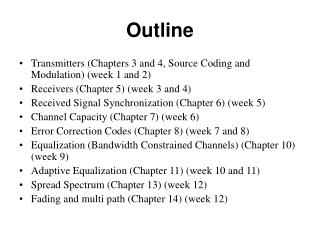DownloadDownload PresentationOutline

# Outline

Télécharger la présentation## Outline

- - - - - - - - - - - - - - - - - - - - - - - - - - - E N D - - - - - - - - - - - - - - - - - - - - - - - - - - -
##### Presentation Transcript

1. Outline • Transmitters (Chapters 3 and 4, Source Coding and Modulation) (week 1 and 2) • Receivers (Chapter 5) (week 3 and 4) • Received Signal Synchronization (Chapter 6) (week 5) • Channel Capacity (Chapter 7) (week 6) • Error Correction Codes (Chapter 8) (week 7 and 8) • Equalization (Bandwidth Constrained Channels) (Chapter 10) (week 9) • Adaptive Equalization (Chapter 11) (week 10 and 11) • Spread Spectrum (Chapter 13) (week 12) • Fading and multi path (Chapter 14) (week 12)

2. Digital Communication System: Information per bit increases Bandwidth efficiency increases noise immunity increases Transmitter Receiver

3. Transmitters (week 1 and 2) • Information Measures • Vector Quantization • Delta Modulation • QAM

4. Receivers (Chapter 5) (week 3 and 4) • Optimal Receivers • Probability of Error

5. Received Signal Synchronization (Chapter 6) (week 5 and 6) • Receivers • Carrier Phase Estimation • Phase Locked Loops • Decision Directed Loops • Symbol Timing Estimation

6. Channel Capacity (Chapter 7) (week 7) • Discrete Memoryless Channels

7. Error Correction Codes (Chapter 8) • Trellis/Convolution Codes • Trellis Codes and QAM NRZI code with memory L=1 Trellis for NRZI Convolution Code Generator Viterbi Algorithm QAM set partition via convolution code 8 state Trellis for rectangular QAM

8. Signals for Band Limited Channels (Chapter 9) (week 10) • Raised cosine

9. Equalization (Chapter 10 and 11) (week 11 and 12) • Receivers for Intersymbol Interference • Decision Feedback Equalization • LMS Algorithm • Adaptive Decision Feedback Equalizer